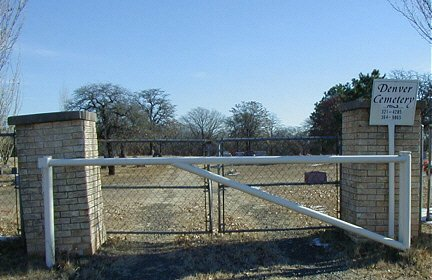### Denver Cemetery

Norman, Cleveland Co., OklahomaThe cemetery is located as follows: From Hwy 9 east of Norman, take 108th Ave SE north about 1.4 miles. The cemetery is on the east (right) side of the road. The names are listed by North, Middle or South section, then Row and within rows the low number is at the north end of the row. Example: N-R1-1 is North Section, Row 1 and the first grave at the north end of the row. M-R5-6 is the sixth grave from the north end of Row 5 of the Middle Section.

Submitted by Jim Woodruff

Cemetery Layout & Directions

Alphabetical
A-B C-G H-N O-T V-Z

list by Lot No.

 Denver Cemetery Layout North East South N-R20 M-R20 S-R20 N-R19 M-R19 S-R19 N-R18 Road M-R18 Road S-R18 N-R17 M-R17 S-R17 N-R16 M-R16 S-R16 N-R15 M-R15 S-R15 N-R14 M-R14 S-R14 N-R13 M-R13 S-R13 N-R12 M-R12 S-R12 N-R11 M-R11 S-R11 N-R10 M-R10 S-R10 N-R9 M-R9 S-R9 N-R8 M-R8 S-R8 N-R7 M-R7 S-R7 N-R6 M-R6 S-R6 N-R5 M-R5 S-R5 N-R4 M-R4 S-R4 N-R3 M-R3 S-R3 N-R2 M-R2 S-R2 N-R1 M-R1 S-R1 West 108th Ave. SE